# Plotting and Visualization

Create 2D and 3D plots and control their style and appearance.

## Functionality

Call the correspondent plot command with the appropriate plotting data as input along with the attributes for controlling the plot appearance and style.
Plot Types and Required Commands

2D and 3D Lines

• plot produces 2D lines.
• plot3 produces 3D lines.

2D and 3D Scatter

• scatter to produces a 2D scatter.
• scatter3 produces a 3D scatter.

2D Contour

contour produces a 2D contour.

3D Contour

countour3 produces a 3D contour.

3D Surface

surf produces a 3D surface.

3D Waterfall

waterfall produces a 3D waterfall.

2D Log Base 10

log produces a 2D plot with log base 10 in both axes.

Polar

polar produces a polar plot.

One or more plots are contained in the Figure window. When you create a plot, a figure window is created as well. You can also create a figure window manually with the figure(n) command:.

The figure(n) command activates the n-th window; the command close(n) closes that same figure. The command close all closes all windows.

## Style and Appearance

Color
• The default colors for the first three lines in a plot are blue, green and red, respectively.
• To control the line and marker color, enter the appropriate character code in a format string after the X, Y and Z input vectors.
Color Code
Blue b
Green g
Red r
Cyan c
Magenta m
Yellow y
Black k
White w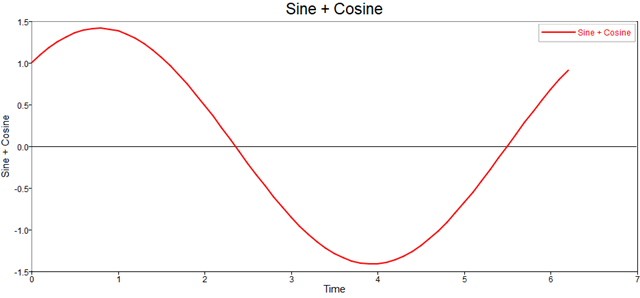Markers
Markers are at the exact locations of the values in the X, Y and Z input vectors. By default, the markers are not shown. To add a marker, enter the appropriate character from the following table to a plot format string.
Coordinate Type Code
Point .
Circle o
x-mark x
Plus +
Star *
Square s
Diamond d
Triangle (down)Triangle (up) ^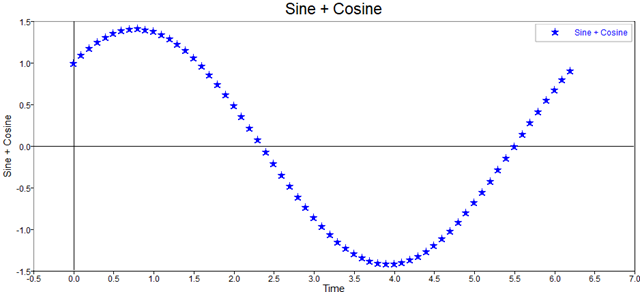## Line Style

The lines within plots are solid by default. To change the line style, add a character from the following table to the data pairs format string.

Line Type Code
Solid -
Dotted :
Dashdot -.
Dashed --
(none) No line is produced.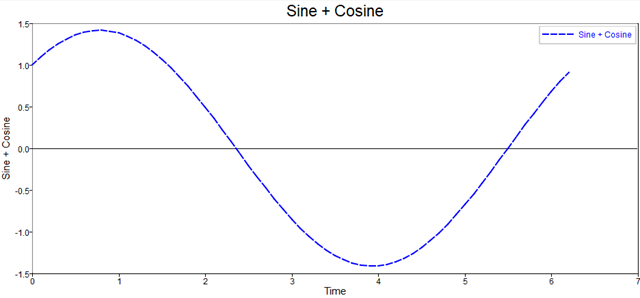Other Functions
• title
• xlabel
• ylabel
• zlabel
• legend
• line
• text
• cla
• clf
• hold
• grid
• axes
• axis
• box
• fill
• hist
• mesh
• xlim
• ylim
• zlim

## Plot Window Management

Define the preference for the windows to be docked or floating: File > Preferences > OML > Plot.

To change the current docking state, select the window docking icon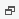on the top border of the figure.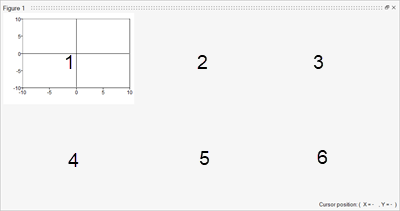The subplot(R, C, N) command creates multiple plots within a single figure window, where:
• R is number of rows of subplots in the window.
• C is number of columns of subplots in the window.
• N is the active plot number.
• and where the plots are numbered from left to right in a row.

## Handles

Plots are considered to be objects in Compose. Each plot object is assigned a numerical value known as a handle. Similarly to object-orientated programming, each object has children that can be modified through the object's handle.

You can modify plots and plot objects programmatically or post plot creation by navigating through the object hierarchy as follows: